# 1669 and Level 6

### Today’s Puzzle:

Some sets of clues in this puzzle have two possible common factors, and another set has three possible common factors. Don’t guess which ones to use, but use logic instead! Write the numbers 1 to 12 in both the first column and the top row so that those numbers and the given clues form a multiplication table.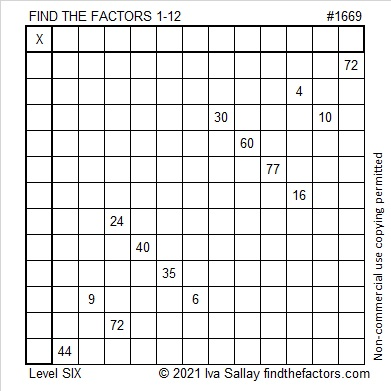### Factors of 1669:

• 1669 is a prime number.
• Prime factorization: 1669 is prime.
• 1669 has no exponents greater than 1 in its prime factorization, so √1669 cannot be simplified.
• The exponent in the prime factorization is 1. Adding one to that exponent we get (1 + 1) = 2. Therefore 1669 has exactly 2 factors.
• The factors of 1669 are outlined with their factor pair partners in the graphic below.How do we know that 1669 is a prime number? If 1669 were not a prime number, then it would be divisible by at least one prime number less than or equal to √1669. Since 1669 cannot be divided evenly by 2, 3, 5, 7, 11, 13, 17, 19, 23, 29, 31, or 37, we know that 1669 is a prime number.

### More About the Number 1669:

1669 is the sum of two squares:
38² + 15² = 1669.

1669 is the hypotenuse of a primitive Pythagorean triple:
1140-1219-1669, calculated from 2(38)(15), 38² – 15², 38² + 15².

Here’s another way we know that 1669 is a prime number: Since its last two digits divided by 4 leave a remainder of 1, and 38² + 15² = 1669 with 38 and 15 having no common prime factors, 1669 will be prime unless it is divisible by a prime number Pythagorean triple hypotenuse less than or equal to √1669. Since 1669 is not divisible by 5, 13, 17, 29, or 37, we know that 1669 is a prime number.

# 1656 Seven Ate Nine: Puzzle and a Picture Book

### Today’s Puzzle:

Today’s Puzzle is a relatively easy level 6 puzzle with consecutive numbers 7, 8, and 9 prominent among the clues. Write the numbers 1 to 10 in both the 1st column and the top row so that those numbers and all the given clues work together to make a multiplication table. Will the common factor of 24 and 32 be 4 or 8? Will 20 and 12’s common factor be 2 or 4? Don’t guess! Look at the other clues. They all work together to help you find a logical way to solve the puzzle.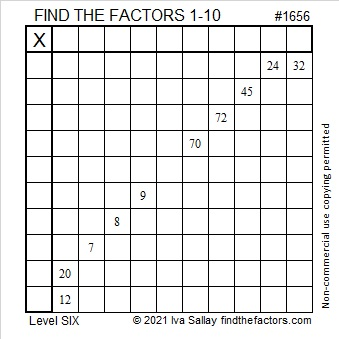### The Book Seven Ate Nine:

I ordered several books from my granddaughter’s book order. One of those books was Seven Ate Nine, a delightful tale whose characters are numbers and letters. The back cover summaries the story, “6 has a problem. Everyone knows that 7 is always after him. Word on the street is that 7 ate 9. If that’s true, 6’s days are numbered. Lucky for him, Private I is on the case. But the facts just don’t add up. It’s odd. Will Private I put two and two together and solve the problem . . . or is 6 next in line to be subtracted?”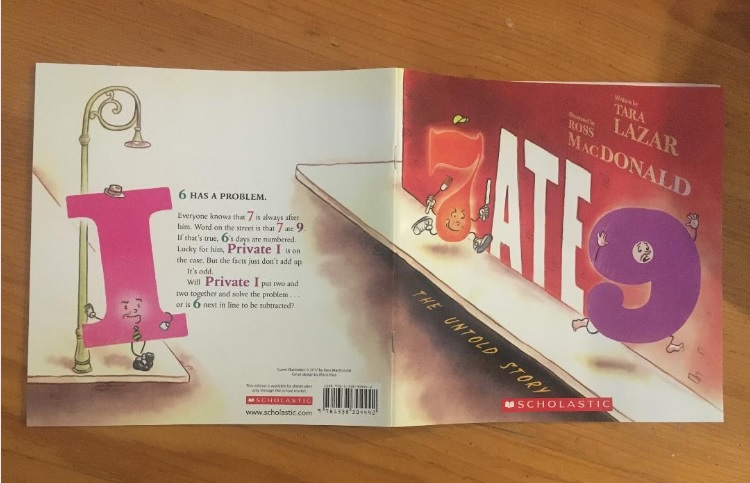My preschool grandchildren loved listening to this story. It is filled with math puns and surprising twists and turns. Other than familiarity with the concept of counting, mathematical understanding is not a prerequisite to following the story. Older kids and even adults will enjoy references to several mathematical concepts including odd, even, addition, subtraction, multiplication, division, doubling, measurement, positives, negatives, and pi.

### Factors of 1656:

• 1656 is a composite number.
• Prime factorization: 1656 = 2 × 2 × 2 × 3 × 3 × 23, which can be written 1656 = 2³ × 3² × 23.
• 1656 has at least one exponent greater than 1 in its prime factorization so √1656 can be simplified. Taking the factor pair from the factor pair table below with the largest square number factor, we get √1656 = (√36)(√46) = 6√46.
• The exponents in the prime factorization are 3, 2, and 1. Adding one to each exponent and multiplying we get (3 + 1)(2 + 1)(1 + 1) = 4 × 3 × 2 = 24. Therefore 1656 has exactly 24 factors.
• The factors of 1656 are outlined with their factor pair partners in the graphic below.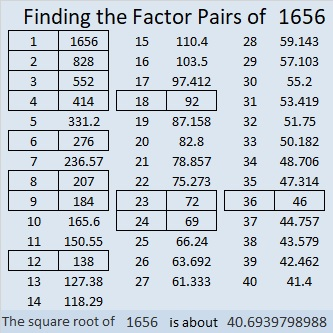### More About the Number 1656:

1656 is the difference of two squares SIX different ways:
415² – 413² = 1656,
209² – 205² = 1656,
141² – 135² = 1656,
75² – 63² = 1656,
55² – 37² = 1656, and
41² – 5² – = 1656.
That last one means we are only 25 numbers away from the next perfect square, 1681.

# 1645 and Level 6

### Today’s Puzzle:

The number 36 appears as a clue in this puzzle three times. None of those 36’s will be 3 × 12 because 21 and 33 must use both 3’s. That means two of the 36’s will be 4 × 9, and one of them will be 6 × 6. Can both of the 36’s associated with the 24 be 4 × 9? Answering that question will help you find the logic needed to know which common factor to use for 72 and 36.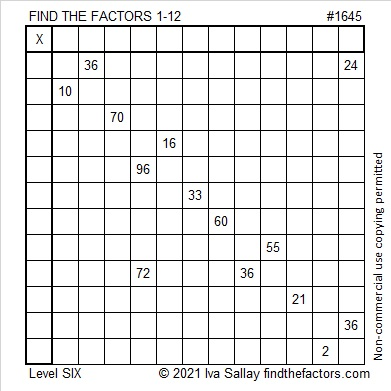### Factors of 1645:

• 1645 is a composite number.
• Prime factorization: 1645 = 5 × 7 × 47.
• 1645 has no exponents greater than 1 in its prime factorization, so √1645 cannot be simplified.
• The exponents in the prime factorization are 1, 1, and 1. Adding one to each exponent and multiplying we get (1 + 1)(1 + 1)(1 + 1) = 2 × 2 × 2 = 8. Therefore 1645 has exactly 8 factors.
• The factors of 1645 are outlined with their factor pair partners in the graphic below.### More About the Number 1645:

1645 is the hypotenuse of a Pythagorean triple:
987-1316-1645, which is (3-4-5) times 329.

# 1634 Be Prepared for April Showers

### Today’s Puzzle:

If you learn the multiplication and division facts in a standard multiplication table, you will be prepared to solve this somewhat tricky April Shower puzzle. You will also be able to solve MANY other mathematical challenges. Use logic to solve it, not guess and check, and it will be much less challenging to find the missing factors.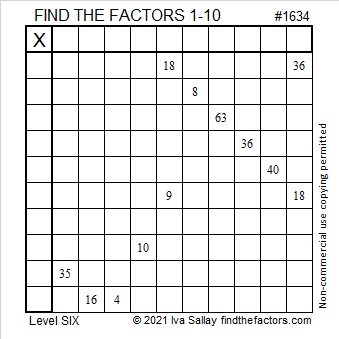### Factors of 1634:

• 1634 is a composite number.
• Prime factorization: 1634 = 2 × 19 × 43.
• 1634 has no exponents greater than 1 in its prime factorization, so √1634 cannot be simplified.
• The exponents in the prime factorization are 1, 1, and 1. Adding one to each exponent and multiplying we get (1 + 1)(1 + 1)(1 + 1) = 2 × 2 × 2 = 8. Therefore 1634 has exactly 8 factors.
• The factors of 1634 are outlined with their factor pair partners in the graphic below.### More about the Number 1634:

1634 is part of exactly two Pythagorean triples. Here are the formulas you can use to calculate those two triples:
2(817)(1), 817² – 1², 817² + 1, and
2(43)(19), 43² – 19², 43² + 19².

Do you see the factors of 1634 prominently displayed in those formulas?

# 1610 Four-Leaf Clovers

### Today’s Puzzle:

Sometimes four-leaf clovers are associated with Saint Patrick’s Day. Four-leaf clovers are supposed to be lucky, but you might not feel so lucky as you work on solving this puzzle. I assure you, there is a logical way to proceed on each step!Print the puzzles or type the solution in this excel file: 14 Factors 1604-1612.

### Factors of 1610:

• 1610 is a composite number.
• Prime factorization: 1610 = 2 × 5 × 7 × 23.
• 1610 has no exponents greater than 1 in its prime factorization, so √1610 cannot be simplified.
• The exponents in the prime factorization are 1, 1, 1, and 1. Adding one to each exponent and multiplying we get (1 + 1)(1 + 1)(1 + 1)(1 + 1) = 2 × 2 × 2 × 2 = 16. Therefore 1610 has exactly 16 factors.
• The factors of 1610 are outlined with their factor pair partners in the graphic below.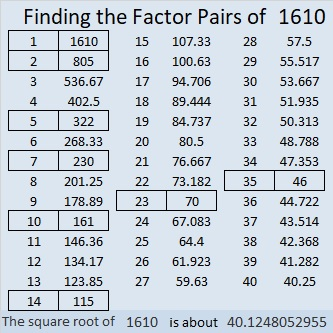### More about the Number 1610:

1610 is the hypotenuse of a Pythagorean triple:
966-1288-1610, which is (3-4-5) times 322.

1610 is not the sum of two squares or the difference of two squares. 1610 is a leg in some Pythagorean triples because
2(805)(1) = 1610,
2(161)(5) = 1610,
2(115)(7) = 1610, and
2(35)(23) = 1610.

You can calculate those Pythagorean triples by letting a be the first number in parenthesis for each of those equations, and b be the second number in parenthesis. Then substitute those values in the three expressions below, and you will have some Pythagorean triples!
2(a)(b), a² – b², a² + b².

# 1601 and Level 6

### Today’s Puzzle:

Remember to use logic for EVERY step when solving this puzzle. Guessing and checking will likely just frustrate you! It’s a level 6 puzzle, so it could be tricky.
Keep in mind:
1 and 2 are common factors of 6 and 8,
3 and 9 are common factors of 9 and 27,
4 & 8 are common factors of 32 and 8, and
4, 5, & 10 are common factors of 20 & 40.

As always, there is only one solution.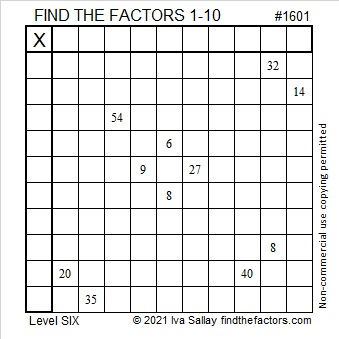### Factors of 1601:

• 1601 is a prime number.
• Prime factorization: 1601 is prime.
• 1601 has no exponents greater than 1 in its prime factorization, so √1601 cannot be simplified.
• The exponent in the prime factorization is 1. Adding one to that exponent we get (1 + 1) = 2. Therefore 1601 has exactly 2 factors.
• The factors of 1601 are outlined with their factor pair partners in the graphic below.

How do we know that 1601 is a prime number? If 1601 were not a prime number, then it would be divisible by at least one prime number less than or equal to √1601. Since 1601 cannot be divided evenly by 2, 3, 5, 7, 11, 13, 17, 19, 23, 29, 31, or 37, we know that 1601 is a prime number.### More about the Number 1601:

1601 is one more than a perfect square, so it is the sum of two squares:
40² + 1² = 1601.

1601 is the hypotenuse of a Pythagorean triple:
80-1599-1601, calculated from 2(40)(1), 40² – 1², 40² + 1².

Here’s another way we know that 1601 is a prime number: Since its last two digits divided by 4 leave a remainder of 1, and 40² + 1² = 1601 with 40 and 1 having no common prime factors, 1601 will be prime unless it is divisible by a prime number Pythagorean triple hypotenuse less than or equal to √1601. Since 1601 is not divisible by 5, 13, 17, 29, or 37, we know that 1601 is a prime number.

# 1591 Conversation Heart

### Today’s Puzzle:

If this valentine-shaped level 6 puzzle gets kids talking about multiplication, then it will truly be a conversation heart.### Factors of 1591:

• 1591 is a composite number.
• Prime factorization: 1591 = 37 × 43.
• 1591 has no exponents greater than 1 in its prime factorization, so √1591 cannot be simplified.
• The exponents in the prime factorization are 1 and 1. Adding one to each exponent and multiplying we get (1 + 1)(1 + 1) = 2 × 2 = 4. Therefore 1591 has exactly 4 factors.
• The factors of 1591 are outlined with their factor pair partners in the graphic below.### More about the Number 1591:

1591 is the difference of two squares two different ways:
796² – 795² = 1591, and
40² – 3² = 1591. That means we are only 3², or 9 numbers away from the next perfect square, 40², or 1600.

# 1582 Half the Time You’ll Be Thinking about Multiples instead of Factors

### Today’s Puzzle:

In the level 6 puzzle, the possible common factors of 40 and 20 are 4, 5, and 10 while the possible common factors of 24 and 12 are 3, 4, and 6.

Don’t worry about which common factor to choose to start the puzzle. Instead, think about multiples. One of the numbers from 1 to 10 will only be able to go in one place in the top row because that’s the only place one of its multiples is in the column below it. That same number will also have only one place it can go in the first column.

You will likely look for lone multiples of particular numbers a total of five times as you solve this puzzle. Good luck!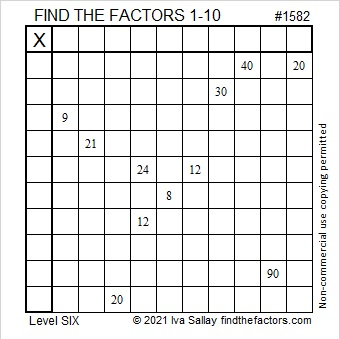### Factors of 1582:

• 1582 is a composite number.
• Prime factorization: 1582 = 2 × 7 × 113.
• 1582 has no exponents greater than 1 in its prime factorization, so √1582 cannot be simplified.
• The exponents in the prime factorization are 1, 1, and 1. Adding one to each exponent and multiplying we get (1 + 1)(1 + 1)(1 + 1) = 2 × 2 × 2 = 8. Therefore 1582 has exactly 8 factors.
• The factors of 1582 are outlined with their factor pair partners in the graphic below.### More about the Number 1582:

1582 is the hypotenuse of a Pythagorean triple:
210-1568-1582, which is 14 times (15-112-113).

1582 is the sum of the four numbers from 394 to 397.

1582 is the sum of the seven numbers from 223 to 229.

1582 is the sum of the twenty-eight numbers from 43 to 70.

# 1564 Two Candles

### Today’s Puzzle:

Candles that are lit in the darkness can be seen from quite a distance away.  Candles and candlelight are symbols of Christmas. The babe born on that first Christmas day would become the Light of the World.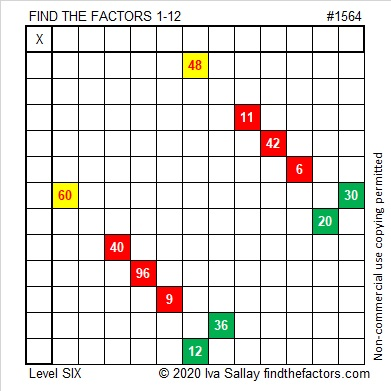What makes a level 6 puzzle more difficult? Can you see that the common factor of 60 and 30 might be 5, 6, or 10? Which one should you use? The other two won’t work with the other clues in the puzzle.

Likewise, the common factor of 48 and 12 might be 4, 6, or 12. Don’t guess which one to use! Use logic, and find the solution to this puzzle.

One blank row and one blank column intersect in a single cell. Can you determine what number belongs in that cell before you write any other factors? That is the first thing I would do.

Here is the same puzzle without any added color: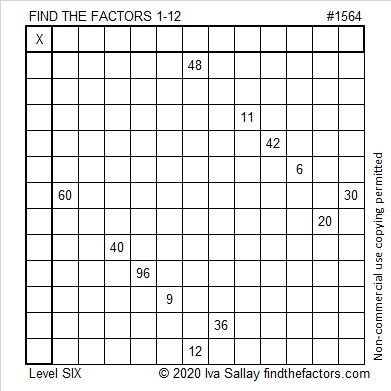### Factor Tree for 1564:

64 is divisible by 4, so 1564 is also. Here is a factor tree for 1564 that divisibility fact:### Factors of 1564:

• 1564 is a composite number.
• Prime factorization: 1564 = 2 × 2 × 17 × 23, which can be written 1564 = 2² × 17 × 23.
• 1564 has at least one exponent greater than 1 in its prime factorization so √1564 can be simplified. Taking the factor pair from the factor pair table below with the largest square number factor, we get √1564 = (√4)(√391) = 2√391.
• The exponents in the prime factorization are 2, 1, and 1. Adding one to each exponent and multiplying we get (2 + 1)(1 + 1)(1 + 1) = 3 × 2 × 2 = 12. Therefore 1564 has exactly 12 factors.
• The factors of 1564 are outlined with their factor pair partners in the graphic below.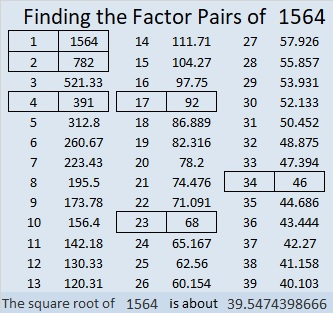### More about the Number 1564:

1564 is the hypotenuse of a Pythagorean triple:
736-1380-1564, which is (8-15-17) times 92.

1564 is the difference of two squares in two different ways:
392² – 390² = 1564, and
40² – 6² = 1564. That means we are only 36 numbers away from 40² = 1600.

1564 is in this cool pattern:# 1553 Ornamental Corn

### Today’s Puzzle:

Ornamental corn is a popular decoration at Thanksgiving. Today’s puzzle looks a little bit like ornamental corn, and there’s at least a kernel of truth to that statement! Solve the puzzle, and I will think YOU are a-maize-ing!Here’s the same puzzle if you want to print it in black and white:### Factors of 1553:

• 1553 is a prime number.
• Prime factorization: 1553 is prime.
• 1553 has no exponents greater than 1 in its prime factorization, so √1553 cannot be simplified.
• The exponent in the prime factorization is 1. Adding one to that exponent we get (1 + 1) = 2. Therefore 1553 has exactly 2 factors.
• The factors of 1553 are outlined with their factor pair partners in the graphic below.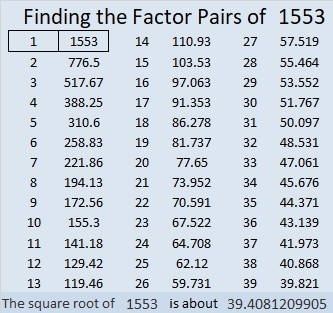How do we know that 1553 is a prime number? If 1553 were not a prime number, then it would be divisible by at least one prime number less than or equal to √1553. Since 1553 cannot be divided evenly by 2, 3, 5, 7, 11, 13, 17, 19, 23, 29, 31, or 37, we know that 1553 is a prime number.

### More About the Number 1553:

1553 is the sum of the squares of two numbers that are reverses of each other:
32² + 23² = 1553

1553 is the hypotenuse of a primitive Pythagorean triple:
495-1472-1553, calculated from 32² – 23², 2(32)(23), 32² + 23².

Here’s another way we know that 1553 is a prime number: Since its last two digits divided by 4 leave a remainder of 1, and 32² + 23² = 1553 with 32 and 23 having no common prime factors, 1553 will be prime unless it is divisible by a prime number Pythagorean triple hypotenuse less than or equal to √1553. Since 1553 is not divisible by 5, 13, 17, 29, or 37, we know that 1553 is a prime number.